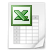High Returns From Low Risk 1929 - 2021 - updated April 2022
-
High Returns from Low Risk - Dataset 192
Microsoft Excel Table 2.8 MB

This dataset file contains two volatility-sorted datasets going back to 1929.

1. The ‘Conservative Formula’

The single-factor decile volatility-sorted portfolios are based on the past 36-months return volatility. The 10 portfolios are equally weighted and constructed at the end of each quarter and include the largest 1,000 NYSE, AMEX, and NASDAQ stocks. The multi-factor ‘conservative formula’ portfolio selects within the 500 lowest-volatility stocks, the 100 stocks with highest net payout ratio and positive price momentum. The Conservative Formula is presented in the book 'High Returns from Low Risk: A Remarkable Stock Market Paradox’ and rigorously tested in the article ‘The Conservative Formula: Quantitative Investing made easy’.

2. The ‘VOL’ factor premium

The long-short volatility factor portfolio is constructed using portfolios based on size and volatility. The double-sorted portfolios are value-weighted and constructed each month and include all NYSE, AMEX and NASDAQ stocks. The portfolio is based on the intersections of two portfolios formed on market equity and three portfolios formed on the past 36-month return volatility. The market equity breakpoint is the median NYSE market equity, and the volatility breakpoints are the 30th and the 70th NYSE percentiles following the Fama-French factor methodology. The VOL factor is market beta-neutral.